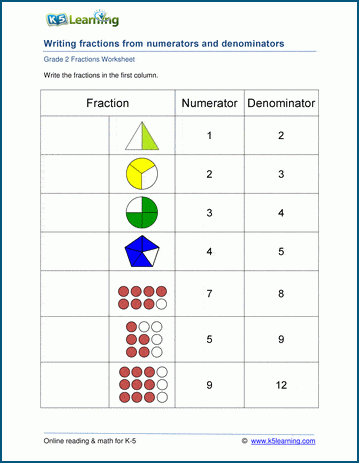# Writing fractions

## Writing fractions in standard form worksheets

In these grade 2 fractions worksheets students are given a numerator, a denominator and an image of a basic fraction, and asked to write the fraction in standard form (i.e. numerator on top, denominator on the bottom).## More fractions worksheets

Explore all of our fractions worksheets, from dividing shapes into "equal parts" to multiplying and dividing improper fractions and mixed numbers.

## What is K5?

K5 Learning offers reading and math worksheets, workbooks and an online reading and math program for kids in kindergarten to grade 5.  We help your children build good study habits and excel in school.